: Data types : Package files in the : Package files in the   目次

## Examples: gb, rrank, gkz, bfunction, deRham

Execute Loadall to load packages before executing examples. Dx means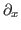.

Example 1   Compute a Gröbner basis and the initial ideal with respect to the weight vector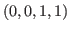of the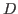-ideal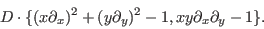See  on the notion of Gröbner basis and the initial ideal with respect to a weight vector.
 [ [( (x Dx)^2 + (y Dy)^2 -1) ( x y Dx Dy -1)] (x,y)
[ [ (Dx) 1 (Dy) 1] ] ] gb pmat ;

Output:
 [
[ x^2*Dx^2+y^2*Dy^2+x*Dx+y*Dy-1 , x*y*Dx*Dy-1 , y^3*Dy^3+3*y^2*Dy^2+x*Dx ]
[ x^2*Dx^2+y^2*Dy^2 , x*y*Dx*Dy , y^3*Dy^3 ]
]

The first line is the Gröbner basis and the second line is a set of generators of the initial ideal with respect to the weight vector. In order to get syzygies, use syz.

Example 2   Generate the GKZ system for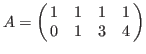and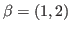. Here, the GKZ system is a holonomic system of differential equations introduced by Gel'fand, Kapranov and Zelevinsky. The system is also called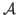-hypergeometric system.
   [ [[1 1 1 1] [0 1 3 4]] [1 2]] gkz  ::

Output:
 [ x1*Dx1+x2*Dx2+x3*Dx3+x4*Dx4-1 , x2*Dx2+3*x3*Dx3+4*x4*Dx4-2 ,
Dx2*Dx3-Dx1*Dx4 , -Dx1*Dx3^2+Dx2^2*Dx4 , Dx2^3-Dx1^2*Dx3 ,
-Dx3^3+Dx2*Dx4^2 ]


Example 3   Evaluate the holonomic rank of the GKZ systems forandand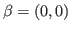. Show also the time of the execution.
  { [ [[1 1 1 1] [0 1 3 4]] [1 2]] gkz  rrank ::} timer
{ [ [[1 1 1 1] [0 1 3 4]] [0 0]] gkz  rrank ::} timer

Output:
   5
User time: 1.000000 seconds, System time: 0.010000 seconds, Real time: 1 s
4
User time: 1.320000 seconds, System time: 0.000000 seconds, Real time: 1 s


Example 4   Compute the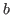-function of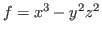and the annihilating ideal of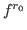where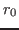is the minimal integral root of the-function.
   (oxasir.sm1) run
[(x^3 - y^2 z^2) (x,y,z)] annfs /ff set
ff message
ff 1 get 1 get fctr ::

Output:
[  [ -y*Dy+z*Dz , 2*x*Dx+3*y*Dy+6 , -2*y*z^2*Dx-3*x^2*Dy ,
-2*y^2*z*Dx-3*x^2*Dz , -2*z^3*Dx*Dz-3*x^2*Dy^2-2*z^2*Dx ]  ,
[-1,-139968*s^7-1119744*s^6-3802464*s^5-7107264*s^4-7898796*s^3-5220720*s^2-1900500*s-294000]]
[[ -12 , 1 ] , [ s+1 , 1 ], [3*s+5 , 1], [ 3*s+4, 1], [6*s+7, 2], [6*s+5, 2]]

The first two rows of the output give generators of the annihilating ideal of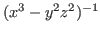. The-function is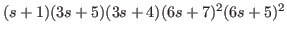and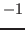is the minimal integral root.

Example 5   Compute the de Rham cohomology group of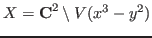.
    (cohom.sm1) run
[(x^3-y^2) (x,y)] deRham ;

Output:
  0-th cohomology:  [    0 , [   ]  ]
-1-th cohomology:  [    1 , [   ]  ]
-2-th cohomology:  [    1 , [   ]  ]
[1 , 1 , 0 ]

This means that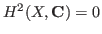,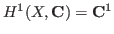,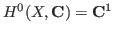.

Example 6   Compute the integral of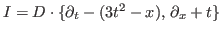, which annihilates the function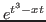, with respect to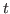.
 (cohom.sm1) run
[ [(Dt - (3 t^2-x)) (Dx + t)] [(t)]
[ [(t) (x)] [ ]] 0] integration

Output:
[    [    1 , [    3*Dx^2-x ]  ]  ]: Data types : Package files in the : Package files in the   目次
Nobuki Takayama 平成22年2月8日